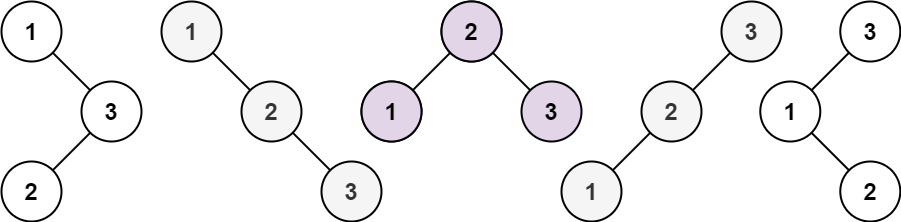# GeetCode Hub

Given an integer `n`, return the number of structurally unique BST's (binary search trees) which has exactly `n` nodes of unique values from `1` to `n`.

Example 1:```Input: n = 3
Output: 5
```

Example 2:

```Input: n = 1
Output: 1
```

Constraints:

• `1 <= n <= 19`

class Solution { public int numTrees(int n) { } }